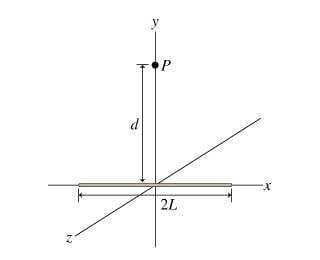Electrostatics/Electric Field Question

Homework Statement

What is the magnitude E of the electric field at point P? Throughout this part, express your answers in terms of the constant k, defined by k = 1/(4πE0)

To solve this question, I am trying to walk through the hint process.

Question 1: Find the magnitude dE of the electric field at point P produced by the infinitesimal wire segment between x and x + dx. Express in terms of λ, d, dx, x, and the constant k.

Question 2: What is dEy, the y component of the electric field at point P due to an infinitesimal segment of wire located at position x? Assume that the magnitude of the electric field at point P due to the infinitesimal wire segment is dE. Express in terms of dE, x, and d.Question 3:
Find dEy(x), the y component of the electric field produced at point P by the infinitesimal segment of the wire between x and x+dx.
Express your answer in terms of x, d, dx, λ, and k.

Homework Equations

E = F/q0 = kq/(r^2) = λ/(2πE0R)
dE = kdq/(r^2) * rhat
|r| = √(x^2 +d^2)
dq = λdx

The Attempt at a Solution

At the moment, I am stuck on question 1.
I tried plugging in my know values but I am unsure of how to express rhat

Since the distance between x and x+dx is dx...

dE = ((kλdx)/(dx^2 +d^2)) * ( (√dx^2+d^2) / √(x^2+d^2) )

Is this the correct formula for dE? What am I assuming wrong?

TSny
Homework Helper
Gold Member

The Attempt at a Solution

At the moment, I am stuck on question 1.
I tried plugging in my know values but I am unsure of how to express rhat

Since the distance between x and x+dx is dx...

dE = ((kλdx)/(dx^2 +d^2)) * ( (√dx^2+d^2) / √(x^2+d^2) )

Is this the correct formula for dE?

No, this is not correct. Please explain how you arrived at this formula.